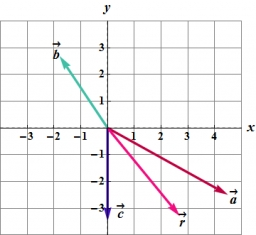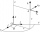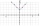# Scalar product

Calculate the scalar product of two vectors: (2.5) (-1, -4)

Correct result:

s =  -22

#### Solution:Our examples were largely sent or created by pupils and students themselves. Therefore, we would be pleased if you could send us any errors you found, spelling mistakes, or rephasing the example. Thank you!

Please write to us with your comment on the math problem or ask something. Thank you for helping each other - students, teachers, parents, and problem authors.Tips to related online calculators
Two vectors given by its magnitudes and by included angle can be added by our vector sum calculator.

## Next similar math problems:

• Angle of the body diagonalsUsing vector dot product calculate the angle of the body diagonals of the cube.
• TriangleTriangle KLM is given by plane coordinates of vertices: K[11, -10] L[10, 12] M[1, 3]. Calculate its area and its interior angles.
• Parametric formCalculate the distance of point A [2,1] from the line p: X = -1 + 3 t Y = 5-4 t Line p has a parametric form of the line equation. ..
• Three points 2The three points A(3, 8), B(6, 2) and C(10, 2). The point D is such that the line DA is perpendicular to AB and DC is parallel to AB. Calculate the coordinates of D.
• Coordinates of square verticesThe ABCD square has the center S [−3, −2] and the vertex A [1, −3]. Find the coordinates of the other vertices of the square.
• Find the 5Find the equation with center at (1,20) which touches the line 8x+5y-19=0
• Vector v4Find the vector v4 perpendicular to vectors v1 = (1, 1, 1, -1), v2 = (1, 1, -1, 1) and v3 = (0, 0, 1, 1)
• Decide 2Decide whether points A[-2, -5], B[4, 3] and C[16, -1] lie on the same line
• Vector perpendicularFind the vector a = (2, y, z) so that a⊥ b and a ⊥ c where b = (-1, 4, 2) and c = (3, -3, -1)
• Vector equationLet’s v = (1, 2, 1), u = (0, -1, 3) and w = (1, 0, 7) . Solve the vector equation c1 v + c2 u + c3 w = 0 for variables c1 c2, c3 and decide weather v, u and w are linear dependent or independent
• Parallel and orthogonalI need math help in this problem: a=(-5, 5 3) b=(-2,-4,-5) (they are vectors) Decompose the vector b into b=v+w where v is parallel to a and w is orthogonal to a, find v and w
• Find the 10Find the value of t if 2tx+5y-6=0 and 5x-4y+8=0 are perpendicular, parallel, what angle does each of the lines make with the x-axis, find the angle between the lines?
• CuboidsTwo separate cuboids with different orientation in space. Determine the angle between them, knowing the direction cosine matrix for each separate cuboid. u1=(0.62955056, 0.094432584, 0.77119944) u2=(0.14484653, 0.9208101, 0.36211633)
• Angle between vectorsFind the angle between the given vectors to the nearest tenth of a degree. u = (-22, 11) and v = (16, 20)
• Calculate 6Calculate the distance of a point A[0, 2] from a line passing through points B[9, 5] and C[1, -1].
• DodecagonCalculate the size of the smaller of the angles determined by lines A1 A4 and A2 A10 in the regular dodecagon A1A2A3. .. A12. Express the result in degrees.
• The modulusFind the modulus of the complex number 2 + 5i